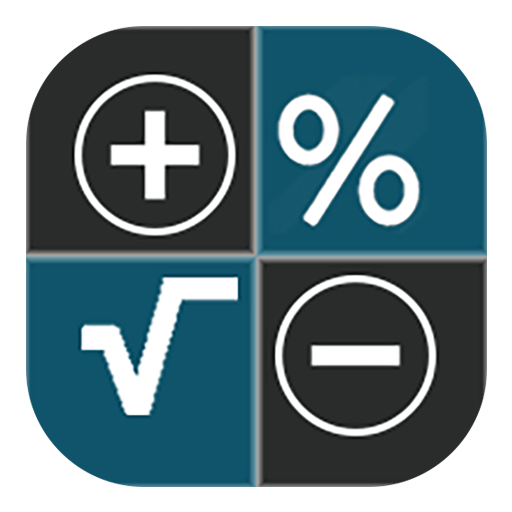# Total Calculator

★ Convenient and smart calculator with built-in function that can use various calculator in
one app and prevent user's mistake as much as possible
★ History, memory storage function, Undo, Redo function
★ Supports 7 different calculator themes
★ Calculator button sound and font change function

- Integrated calculator configuration -

1) Simple Calculator
1) General Calculator
2) Engineering Calculator
3) Statistical calculator
4) Precision Calculator
5) Date Calculator
6) Unit conversion
7) Percent Calculator
8) Interest calculator (deposit / savings / loan)

★ Free version will show ads.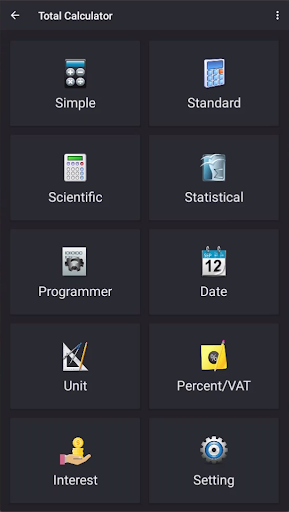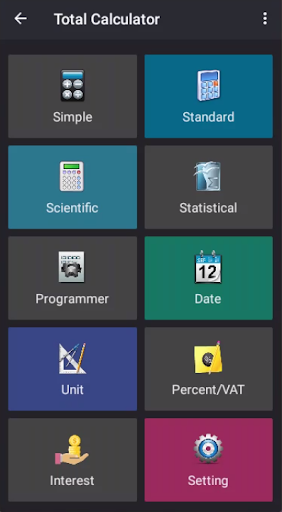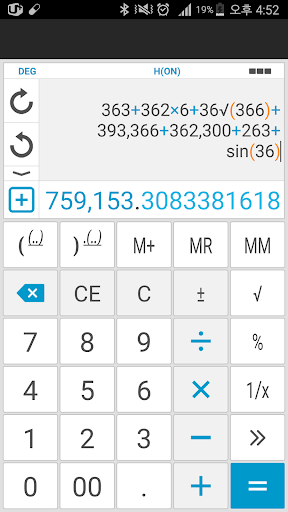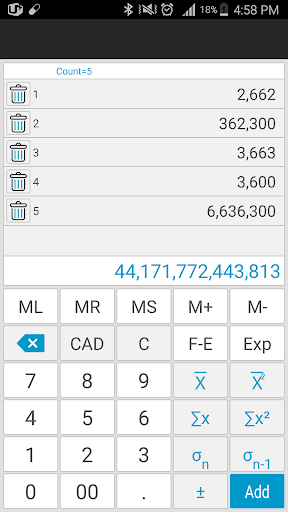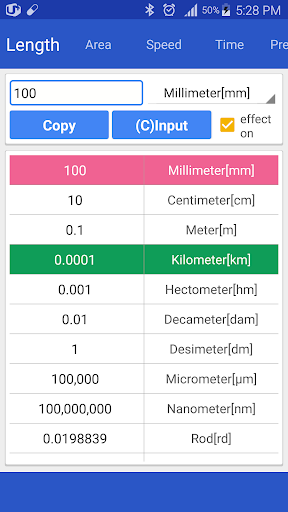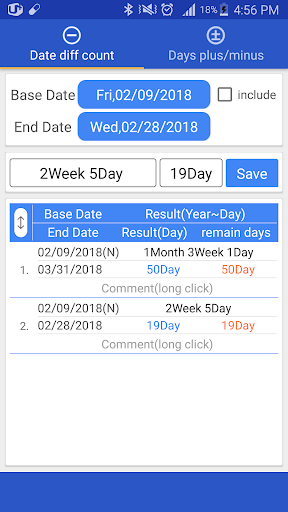##### Recent Updates - Version 15.7
• ☞ ver 15.7
→ Theme error improvement